# 2nd PUC Basic Maths Question Bank Chapter 8 Bill Discounting Ex 8.1

Students can Download Basic Maths Exercise 8.1 Questions and Answers, Notes Pdf, 2nd PUC Basic Maths Question Bank with Answers helps you to revise the complete Karnataka State Board Syllabus and score more marks in your examinations.

## Karnataka 2nd PUC Basic Maths Question Bank Chapter 8 Bill Discounting Ex 8.1

Part – A and B

2nd PUC Basic Maths Bill Discounting Ex 8.1 One or Two Marks Questions with Answers

Question 1.
A bill was drawn on 14-3-2013 for 3 months. When does the bill fall legally due?
L.D.D = DD + BP + 3 days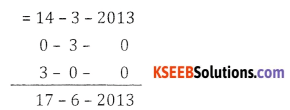∴L.D.D is 17th June 2013Question 2.
A bill drawn for 3 months was legally due on 18-8-2012. Find the date of drawing of the bill.
Drawn date = L.D.D – Bill period – 3days grace = 14 – 3 – 2013
= 18 – 8 – 2012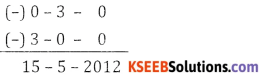∴ Drawn date is 15th May 2012

Question 3.
Find the present value of ₹ 750 due 4 months hence at 15% p.a.
Given
F = 750, T = 4 months = $$\frac{4}{12}$$, r= 15% = 0.15.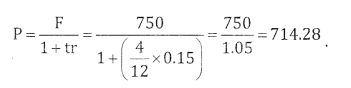Question 4.
Find the banker’s discount on a bill of ₹ 415 due 9 months hence at 15% p.a.
Given
F = 415, t = $$\frac{9}{12}$$ year,
r = 15% = 0.15
B.D = Ftr = 415 x $$\frac{9}{12}$$ × 0.15= 46.68.

Question 5.
Define Banker’s Gain.
Banker gain is difference between BD & TD i.e., BG = BD – TD

Question 6.
Define Banker’s Discount.
Banker’s Discount is the Simple interest calculated by the banker on the face value of the bill i.e., BD = Ftr

Question 7.
Find the present value of 2320, due 2 years hence, at 8% per annum.
Given F= 2320,t = 2years, r = 0.08, P = ?Question 8.
Find the BD on ₹ 1015, payable after 3 months at 6% p.a.
Given
F = 1015,
F=1015, t = $$\frac{3}{12}$$ year, r = $$\frac{6}{100}$$ = 0.06, BD = ?
BD = Ftr = 1015 x $$\frac{3}{12}$$ × 0.06 = 15.22Question 9.
Find the true discount on ₹ 1380, due 1$$\frac{1}{2}$$ years after, at 10% p.a.
Given
F = ₹ 1380, t = $$\frac{3}{2}$$ year , r = 10% = 0.1.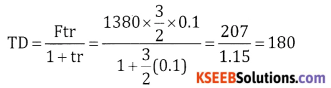Question 10.
TD on a bill was ₹ 100 and BG was ₹ 10. What is the face value of the bill?
Given TD = 100, BG = 10, F = ?
BD = TD + BG = 100 + 10 = 110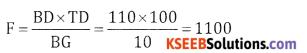Question 11.
A banker pays ₹ 2380 on a bill of ₹ 2500, 73 days before the legal due date. Find the rate of discount charged by the banker.
Given Discounted value = 2380, F = 2500
t = $$\frac{73}{365}=\frac{1}{5}$$ year , r = ?
D.V. = F(1 – tr)
2380 = 2500 $$\left(1-\frac{1}{5} r\right)$$
$$\frac{2380}{2500}=\left(1-\frac{r}{5}\right)$$
0.952 = 1 – $$\frac{r}{5}$$
$$\frac{r}{5}$$ = 1 – 0.952 = 0.048
∴ r = 5 × 0.048 = 0.24 × 100 = 24%

Question 12.
The Banker’s discount and true discount on a sum of money due 3 months hence are ₹ 154.50 and ₹ 150 respectively. Find the sum of money and the rate of interest.
Given BD = 154.5, t = $$\frac{3}{12}$$ , TD = 150, t = ? r = ?Question 13.
BD and BG on a certain bill due after sometime are 1250 and 350 respectively. Find the face value of the bill.
BD and BG on a
Given
BD = 1250, BG = 50, F = ?
BG = BD – TD
TD = BD – BG = 1250 – 50 = 1200Part – C

2nd PUC Basic Maths Bill Discounting Ex 8.1 Three or Four Mark Questions with Answers

Question 1.
The difference between banker’s discount and true discount on a bill due after 6 months at 4% interest p.a. is ₹ 20. Find the true discount, banker’s discount and face value of the bill.
Given
BG = ₹ 20, r = 4% = 0.04, t = 6 Months = $$\frac{6}{12}=\frac{1}{2}$$
TD = ?, BD = ?, F = ?
W.K.T. = BG = TD. tr
20 = TD $$\frac{1}{2}$$ x 0.04
40 = 0.04 TD ⇒ TD = $$\frac{40}{0.04}$$ = ₹ 1000
BD = BG + TD; = 20 + 1000 = 1020Question 2.
The BG on a certain bill due 6 months hence is ₹ 10, the rate of interest being 10% p.a. find the face value of the bill and the true present value.
BG = 10, ⇒ BD – TD = 10,t = $$\frac{6}{12}=\frac{1}{2}$$year, r= 10% = 0.1, F = ? P = ?
BG = TD.tr
10 = TD × $$\frac{1}{2}$$ × 0.1
TD = $$\frac{20}{0.1}$$ = 200 ;
BD = TD + BG = 200 + 10 = 210.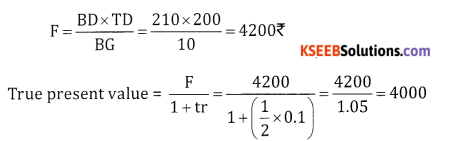Question 3.
A banker discounts a bill for a certain amount having 32 days to run before it matures at 15% p.a. The discounted value of the bill is ₹ 995.90. What is the face value of the bill, banker’s discount, true discount and banker’s gain?
Given t = $$\frac{32}{365}$$ year, r = 0.15, D.V = ₹995.90, F = ?, BD = ?, TD = ?, BG = ?
Discounted Value = F(1 – tr), 995.90 = F ( 1 – $$\frac{32}{365}$$ × 0.05)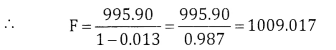D= F – Discounted Value;
= 1009.017 – 995.90 = 13.117BG = BD – TD = 13.117 – 12.948 = 0.169

Question 4.
A bill for ₹ 14,600 drawn at 3 months after date was discounted on 11-11-99 for ₹ 14,320. If the discount rate is 20% p.a. on what date was the bill drawn?
Given DV = 14320, F = 14,600, r=0.2,
DD = ?
W.K. Discounted value = F(1 – tr)
14320 14,320 = 14,600 (1 – 0.2t);
$$\frac{14320}{14600}$$ = 1-0.2t
t = 0.1 year = 0.1 ~ 365 = 36 days
L.D.D = 36 days + 11-11-99 = Dec. 17
Drawn date = L.D.D – BP – 3 grace days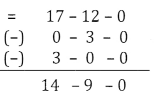∴Bill was drawn on October 14th 3

Question 5.
A bill for ₹ 2920 was drawn on September 11 for 3 months after date and was discounted at 16% p.a. for ₹ 2875.20. On what date was the bill discounted?
Given drawn date = 11th Sep., F = 2920, DV = 2875.20, r = 0.16
BD = F – Discounted value
= 2920 – 2875.20
BD = 44.8
& BD = Ftr.
44.8 = 2920 × 0.16 × t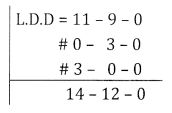t = $$\frac{44.8}{2920 \times 0.16}$$ = 0.0958 years = 0.0958 × 365 = 35 days
Discounted date = L.D.D – Discount period = Dec. 14th – 35 days = 9th NovemberQuestion 6.
A bill for ₹ 3500 due for 3 months was drawn on 27 march 2012 and was discounted on 18 April 2012, at the rate of 7% p.a. Find the banker’s discount and discounted value of the bill.
Given
F = 3500, r = 0.07, B.P = 3 Months
DD = 27th March 2012, Discounted on 18th April 2012; BD = ?, DV = ?
L.D.D = DD + BD + 3 grace days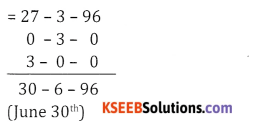t = No. of days from April 18th to 30th June
12 + 31 + 30 = 73 days =$$\frac{73}{365}$$ Year
DV = F(1 – tr) = 3500 1 – $$\frac{73}{365}$$ x 0.07)
DV = 3451.00;
BD = F – DV = 3500 – 3451 = ₹ 49

Question 7.
A bill for 12900 was drawn on 3 Feb 2004 at 6 months and discounted on 13 March 2004 at 8% p.a. For what sum was the bill discounted and how much did the banker gain in this transaction?
Given
F = 12900, r = 0.08, BG = ?
L.D.D = DD + BP + 3days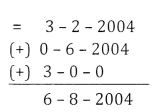t = No. of days from discounted date till LDD = 13 – 3 – 200 to 6 – 8 – 2004
Mar. + Apr. + May + June + July + Aug
= 18 + 30 + 31 + 30 + 31 + 6 = 146 days = $$\frac{146}{865}$$
∴OV = F(1 – tr)
= 12900 (1 – $$\frac{146}{365}$$ x 0.08
= 12900 (1 -0.032)
DV = 12487.2
BD = F – Discounted value
= 12900 – 12487.2 = 412.8
TD = $$\frac{B D}{1+t r}=\frac{412.8}{1+0.032}$$ = 400
BG = BD – TD = 412.8 – 400 = ₹ 12.8

Question 8.
The banker’s gain on a bill is $$\frac{1}{9}$$ th of the banker’s discount, rate of interest being 10% p.a. Find the unexpired period of the bill.
Given BG = $$\frac{1}{9}$$BD & r= 0.1
9(BD – TD) = BD
8BD = 9TD
BD 8BD=9. $$\frac{\mathrm{BD}}{1+\mathrm{tr} \mathrm{r}}$$
1+ tr = $$\frac{9}{8}$$ = 1.125
tr = 1.125 – 1
t(0.1) = 0.125
t = $$\frac{0.125}{0.1}$$ = 1.25years
t = 1.25 × 12 = 15 monthsQuestion 9.
A bill for ₹ 2725.25 was drawn on 03-06-2010 and made payable 3 months after due date. It was discounted on 15-6-2010 at 16% per annum. What is the discounted value of the bill and how much did the banker gain?
= 15 +31 +31 + 6 = 83 days = $$\frac{83}{365}$$ years
= 2725.25 (1 – $$\frac{83}{365}$$ × 0.16 = 2725.25 (1 – 0.036)
TD = $$\frac{B D}{1+t r}=\frac{98.10}{1.036}$$ = 94.69